Physics - Classical Mechanics - Exercises around Pendulums

in STEMGeeks16 days ago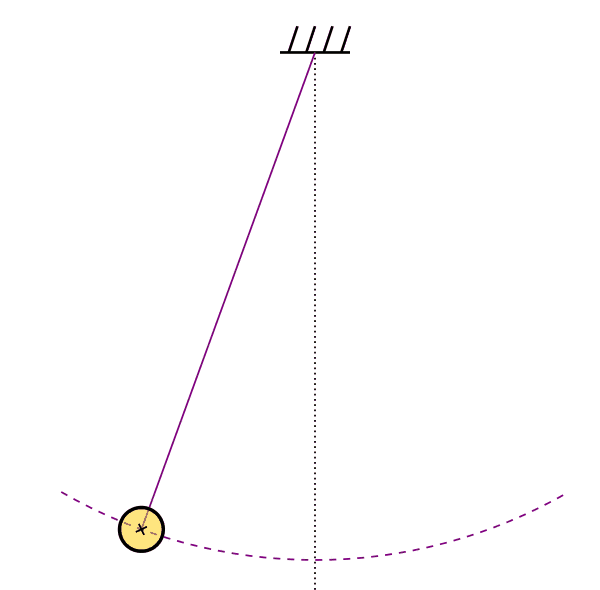[Image1]

Introduction

Hey it's a me again @drifter1!

Today we continue with Physics, and more specifically the branch of "Classical Mechanics" in order to get into Exercises around Pendulums.

So, without further ado, let's get straight into it!

Example 1: Simple Pendulum

Consider a simple pendulum of length L = 4.9 m and maximum angular displacement θ0 = 0.5 rad, as shown in the figure below.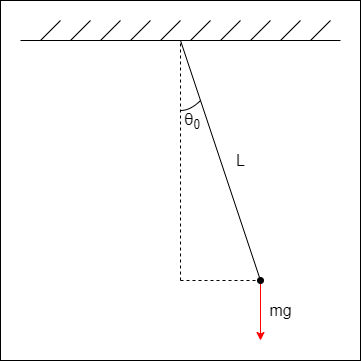Let's:

• calculate the angular frequency (ω), period (T) and frequency (f) of the periodic motion
• write the angular displacement (θ) as a function of time. The initial phase is φ = 0 (maximum positive displacement - as in the figure)
• calculate the maximum "tangent" acceleration (amax)

Note: Approximate simple pendulum motion as SHM by applying the small angle approximation formulas. The acceleration of gravity is g = 9.8 m / s 2.

Solution

Applying small angle approximation, the angular frequency equals: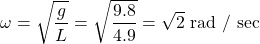And so, the oscillation period and frequency are: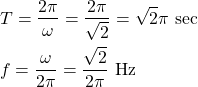The maximum angular displacement is known, and thus writing the angular displacement as a function of time is a simple substitution of the known values: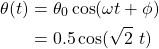The only force acting upon the motion is gravity. Thus, taking the component of gravity which is tangent to the motion, the maximum acceleration equals: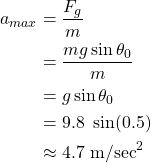The maximum acceleration can also be calculated by taking the square of the angular frequency and multiplying it with the amplitude. Of course, the amplitude in that case is equal to L sinθ0, which is the maximum horizontal displacement, giving us: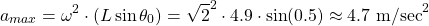With small angle approximation (sinθ ≈ θ) the maximum acceleration would be 4.9 m / s 2 in both cases, which introduces a little bit of error, and so sin(0.5) ≈ 0.48 was used instead!

Continuing On...

In a similar notion, the maximum velocity equals: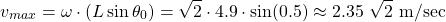And writing the velocity and acceleration as functions of time is as simple as: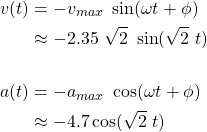Example 2: Physical Pendulum

Let's next consider a uniform rod of length d and mass m, that is pivoted at one end, as shown in the figure below. This physical pendulum starts oscillating with a maximum angular displacement of θ0.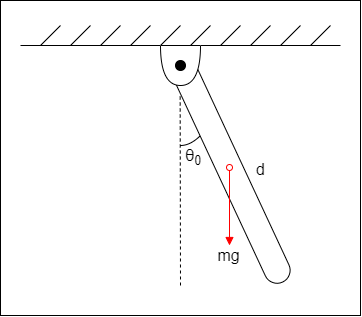Express the angular frequency (ω) and period of oscillation (T) in terms of d and g only. How does the period change when the length of the rod is doubled?

Note: The moment of inertia at the end points of a rod is given by Ip = 1 / 3 md 2. Approximate the physical pendulum oscillation as SHM.

Solution

The center of mass (and gravity) of a uniform rod is exactly at it's center, and so L = d / 2.

Thus, the angular frequency is given by: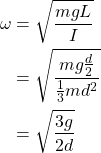and the period by: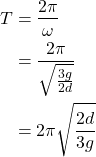For d' = 2d, the corresponding L' equals d, which gives a period of: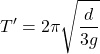which, in turn, relates to the original period as follows: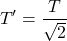Continuing On...

Let's suppose that m = 1 Kg, g = 9.8 m / s 2 and d = 2 m. We can now estimate the moment of inertia by calculating the period (T) and solving the period equation for I. Let's afterwards compare that value to the expected one!

For the given values, the period equals: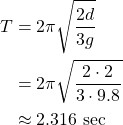and so the moment of inertia is: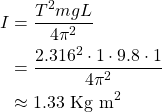The corresponding expected value is: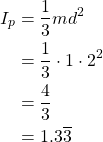The results are very close and so the error can be considered infinitesimal!

RESOURCES:

References

Previous parts...

Final words | Next up

And this is actually it for today's post!

Next time we will get into Damped Oscillation...

See ya!Keep on drifting!

Posted with STEMGeeks

Sort:Thanks for your contribution to the STEMsocial community. Feel free to join us on discord to get to know the rest of us!

Please consider delegating to the @stemsocial account (85% of the curation rewards are returned).

You may also include @stemsocial as a beneficiary of the rewards of this post to get a stronger support.